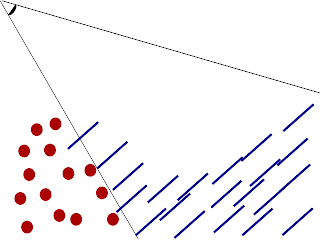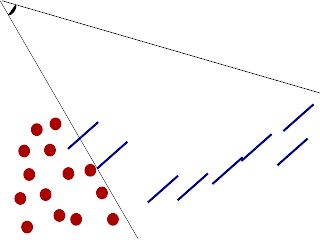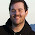## Saturday, April 2, 2011

### Screen Space Fluid rendering Phase 1

As I mentioned in my previous post, I have currently coded three of the necessary phases for rendering a set of points as fluid surface. The technique used is referred to as "Screen Space Fluid Rendering." The three phases are "Render points as Spheres", "Smooth the depth buffer", and finally "Calculate normals from the Smoothed Depth Buffer". In this blog, I will elaborate more on the screen space fluid rendering technique and I will discuss the first step.

Introduction to Screen Space Fluid Rendering(SSFR)

Before going into the details of each phase, I would like to introduce a little theory behind SSFR. The primary goal behind SSFR is to reconstruct the fluid surface in the viewer's (camera's) space. Concerning ourselves with only the viewer's perspective have potential speed up over methods like marching cubes which attempt to reconstruct the fluids entire surface. SSFR is not without limitations but for generating real time fluid animations it is among the fastest and highest quality currently available. An improvement in the smoothing phase, was developed and titled SSFR with Curvature flow.Figure 1: The viewer typically can only see a subset of the particles. Also, they can almost never see the opposite surface. These factors motivate the need for creating the surface in user's perspective only.Figure 2: Points outside the viewer's perspective are clipped. This is another way to save time.Figure 3: The green line represents the surface we hope to obtain by the end phase.
Creating Spheres from points

The first phase is to create spheres from a collection  of points. If we choose to actually render sphere meshes at each point the algorithm would become very expensive as the number of particles grows. Instead we choose to employ a GLSL shader similar to the one found in Nvidia's oclParticle demo. We only need to use a shader because after this phase we no longer use vertex information. All subsequent phases take output from previous phases and process the image.

uniform float pointRadius;
uniform float pointScale;   // scale to calculate size in pixels

varying vec3 posEye;        // position of center in eye space

void main()
{

posEye = vec3(gl_ModelViewMatrix * vec4(gl_Vertex.xyz, 1.0));
float dist = length(posEye);
gl_PointSize = pointRadius * (pointScale / dist);

gl_TexCoord = gl_MultiTexCoord0;
gl_Position = gl_ModelViewProjectionMatrix * vec4(gl_Vertex.xyz, 1.0);

gl_FrontColor = gl_Color;
}


uniform float pointRadius;  // point size in world space
uniform float near;
uniform float far;
varying vec3 posEye;        // position of center in eye space

void main()
{
// calculate normal from texture coordinates
vec3 n;
n.xy = gl_TexCoord.st*vec2(2.0, -2.0) + vec2(-1.0, 1.0);
//This is a more compatible version which works on ATI and Nvidia hardware
//However, This does not work on Apple computers. :/
//n.xy = gl_PointCoord.st*vec2(2.0, -2.0) + vec2(-1.0, 1.0);

float mag = dot(n.xy, n.xy);
if (mag > 1.0) discard;   // kill pixels outside circle
n.z = sqrt(1.0-mag);

// point on surface of sphere in eye space

vec4 clipSpacePos = gl_ProjectionMatrix*spherePosEye;
float normDepth = clipSpacePos.z/clipSpacePos.w;

// Transform into window coordinates coordinates
gl_FragDepth = (((far-near)/2.)*normDepth)+((far+near)/2.);
gl_FragData = gl_Color;
}


NOTE: The fragment shader above has some compatibility issues with ATI cards. Apparently the appropriate way to handle a point sprites texture coordinates is through gl_PointCoord. However this is not compatible with Apples OpenGL implementation.Figure 4: Turn the points from the Figure 2 into point sprites. Point sprites are useful because they always face the viewer.Figure 5: Point sprites which are "below" the surface do not need to be rendered.
The main goal of this shader is to modify the depth values of the rasterized image. To do this we must determine the z value from a 2D texture coordinate. First, take a look at the formula for a sphere.

$x^2+y^2+z^2 = 1$

Notice that we are outside the sphere if the following condition occurs:

$x^2 + y^2 > 0$

...
// calculate normal from texture coordinates
vec3 n;
n.xy = gl_TexCoord.st*vec2(2.0, -2.0) + vec2(-1.0, 1.0);
float mag = dot(n.xy, n.xy);
if (mag > 1.0) discard;   // kill pixels outside circle
...


If we are in the sphere then the following calculation will give us the z value of the point on the sphere.

$z = \sqrt{1-x^2-y^2}$

...
n.z = sqrt(1.0-mag);
...


The z value from this calculation is normalized on the interval [0,1]. The next step is to project this back into 3D camera coordinates. After projecting it into camera coordinates we must manually transform it back into window coordinates.

...
// point on surface of sphere in camera space

vec4 clipSpacePos = gl_ProjectionMatrix*spherePosEye;
float normDepth = clipSpacePos.z/clipSpacePos.w;

// Transform into window coordinates coordinates
gl_FragDepth = (((far-near)/2.)*normDepth)+((far+near)/2.);
...Figure 6: Morph the point sprites into hemispheres.

In my next post, I plan to explain how to modify the depth values in a way to make these bumpy spheres look more like a continuous surface.

All shader code and c++ code are available from enjalot's github repository. Rendering code can be found in rtps/rtpslib/Render/.

1.Hi, I have some doubts about this techinque. Could you help me? I'm trying to calculate de normal of the surface but a get some weird stuff.

Thanks,
Edgar

2.3.I want to attach the depth buffer to a texture2D. Any idea? Thanks.

4.Forget it. I could achieve! Thanks anyway!

5.Happy new year!! :D

How a can compile your project under Windows? Thanks and have fun.

6.Thanks and happy new year to you. :)

If you check out the windows branch from github it should work. However, that version is behind quite a bit from the master branch. But, it should include all the rendering stuff.

BTW, I have forked the repo to my own git hub. https://github.com/ayoung200/EnjaParticles That is where all new development will be. I currently don't have it compiling on windows but it is high on my Todo list.

7.Hi, Could you post a photo or a video? Thanks.

8.My latest post has a video of this in action. http://andrewfsu.blogspot.com/2011_11_01_archive.html The latest version also has the ability to render the velocity vectors of each particle.

9.Hi, What happens if I blur the normal map instead of the depth buffer? I can't get the normal calculation from the depth.

10.I have not tried to blur the normal map. I don't think you will obtain the same results though. If you get my code from github, https://github.com/ayoung200/EnjaParticles , you will find all the code and shaders are available. The shader called normal_vert.glsl and normal_frag.glsl will calculate the normals based on the depth buffer.

11.Hi Again, how are you?

I've been trying some smoothing algorithms but none of them convinces me at all, I want to do the curvature flow technique, so did you implement it? Thanks again

1.You have to have a very large filter width. I use a diameter of 60 here and it produces reasonable results. This algorithm is horribly inefficient at high resolutions because it requires 30^2*height*width operations. More efficient algorithms exists but I haven't had a chance to test them. I tried separable gaussian blur but it had artifacts.

#define KERNEL_DIAMETER 60
const float sigmasq = 64. ;//0.84089642*0.84089642;
const float pi = 3.141592654;

//float kernel[KERNEL_SIZE];

uniform sampler2D depthTex;
uniform float del_x;
uniform float del_y;

float linearizeDepth(float depth, float f, float n)
{
return (2*n)/(f+n-depth*(f-n));
}
float nonlinearDepth(float depth, float f, float n)
{
return ((-2*n)/depth+f+n)/(f-n);
}
//vec2 offset[KERNEL_SIZE];

void main(void)
{
float depth=texture2D(depthTex, gl_TexCoord.st).x;
// float depth=linearizeDepth(texture2D(depthTex, gl_TexCoord.st).x,1000.0,0.3);
float maxDepth=0.999999;
//float maxDepth=1.0;
//float threshold=0.01;
if(depth>maxDepth)
{
//return;
}
float sum = 0.0;
for(int i=0; ithreshold)
// tmp=depth;//+(sign(tmp-depth))*threshold;//continue;
sum += tmp * (1./(2.*pi*sigmasq))*exp(-(pow(float(i-(KERNEL_DIAMETER/2)),2.)+pow(float(j-(KERNEL_DIAMETER/2)),2.))/(2.*sigmasq));
}
}
//if(sum<0.05)
// sum=1.0;
gl_FragData = vec4(sum,sum,sum,1.0);
//sum = nonlinearDepth(sum,1000.0, 0.3);
gl_FragDepth = sum;
}

12.Hi Andrew!

I'm working on a project where I plan to render non photo-realistic water using a SPH fluid simulation, and I'm trying the method you describe in this post get the surface. Right now the application can render point sprites and its normals (https://www.dropbox.com/s/i9njk5sbaxr2wrl/Depth_shader_normals.png), but we have two problems: first, the point sprites seems to be rendered way bigger than the pointRadius we pass as parameter to the shader, and actualy the only way we managed to render it with a smaller radius was to change the value compared with mag at this line: "if (mag > 1.0) discard;" to a lower number, since changing the pointRadius uniform variable don't seems to change anything. And secondly we tried to render the depth buffer (stored in the gl_FragDepth) this way, in the shader itself: gl_FragData = vec4(gl_FragDepth, gl_FragDepth, gl_FragDepth, 1.0), but what it gives to us is the entire particle set rendered in full black (https://www.dropbox.com/s/1uqc8f5sigxm06u/Depth_shader_allBlack.png). We tried to render the calculated distance (dist, from the vertex shader) to, but it seems to be equal for all particles.
Do you have any ideas that maybe can help us?